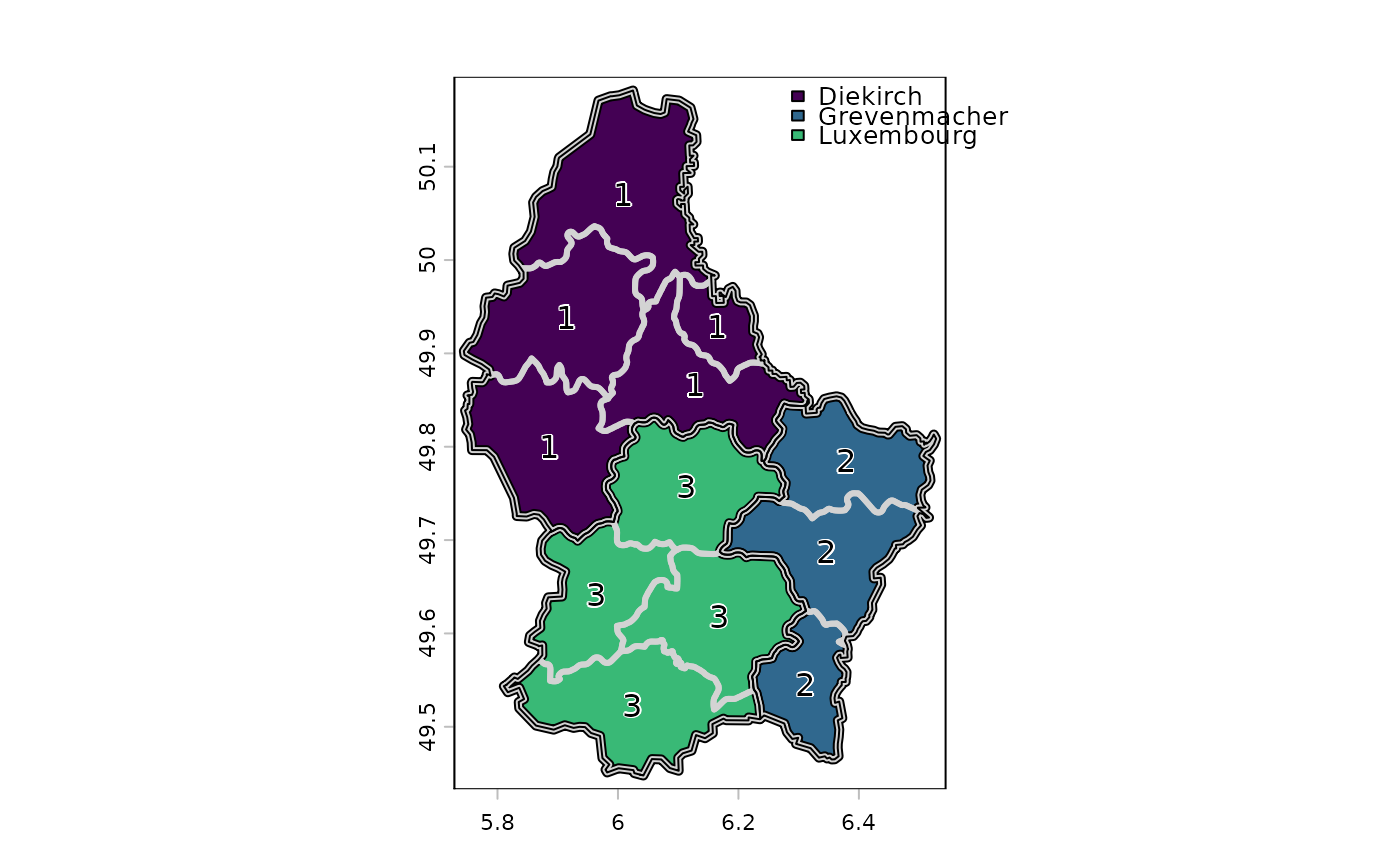Aggregate a SpatRaster to create a new SpatRaster with a lower resolution (larger cells). Aggregation groups rectangular areas to create larger cells. The value for the resulting cells is computed with a user-specified function.

You can also aggregate ("dissolve") a SpatVector. This either combines all geometries into one geometry, or it combines the geometries that have the same value for the variable(s) specified with argument by.

# S4 method for SpatRaster
aggregate(x, fact=2, fun="mean", ..., cores=1, filename="", overwrite=FALSE, wopt=list())

# S4 method for SpatVector
aggregate(x, by=NULL, dissolve=TRUE, fun="mean", count=TRUE, ...)

## Arguments

x

SpatRaster

fact

positive integer. Aggregation factor expressed as number of cells in each direction (horizontally and vertically). Or two integers (horizontal and vertical aggregation factor) or three integers (when also aggregating over layers)

fun

function used to aggregate values. Either an actual function, or for the following, their name: "mean", "max", "min", "median", "sum", "modal", "any", "all", "prod", "which.min", "which.max", "sd" (sample standard deviation) and "std" (population standard deviation)

...

additional arguments passed to fun, such as na.rm=TRUE

cores

positive integer. If cores > 1, a 'parallel' package cluster with that many cores is created. Ignored for C++ level implemented functions that are lister under fun

filename

character. Output filename

overwrite

logical. If TRUE, filename is overwritten

wopt

list with named options for writing files as in writeRaster

by

character. The variable(s) used to group the geometries

dissolve

logical. Should borders between aggregated geometries be dissolved?

count

logical. If TRUE and by is not NULL, a variable "agg_n" is included that shows the number of input geometries for each output geometry

## Details

Aggregation starts at the upper-left end of a SpatRaster. If a division of the number of columns or rows with factor does not return an integer, the extent of the resulting SpatRaster will be somewhat larger then that of the original SpatRaster. For example, if an input SpatRaster has 100 columns, and fact=12, the output SpatRaster will have 9 columns and the maximum x coordinate of the output SpatRaster is also adjusted.

The function fun should take multiple numbers, and return a single number. For example mean, modal, min or max.

It should also accept a na.rm argument (or ignore it as one of the 'dots' arguments).

disagg to disaggregate

SpatRaster

## Examples

r <- rast()
# aggregated SpatRaster, no values
ra <- aggregate(r, fact=10)

values(r) <- runif(ncell(r))
# aggregated raster, max of the values
ra <- aggregate(r, fact=10, fun=max)

# multiple layers
s <- c(r, r*2)
x <- aggregate(s, 20)

## SpatVector
f <- system.file("ex/lux.shp", package="terra")
v <- vect(f)
va <- aggregate(v, "ID_1")

plot(va, "NAME_1", lwd=5, plg=list(x="topright"), mar=rep(2,4))
lines(v, lwd=3, col="light gray")
lines(va)
text(v, "ID_1", halo=TRUE)